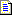English (English)

# Hypotheses and optimizing the search

When the program matches a FlexiLayout with an image, it attempts to find the objects on the image which correspond to the elements in the FlexiLayout. The program then estimates how well a particular object matches its element. An estimate is a number from 0 to 1. A quality of 1 means that the detected object is a 100% match. If the quality is not 0, matching the FlexiLayout results in creating regions for the blocks.

The program looks for elements consecutively in the order in which they follow in the FlexiLayout tree, top to bottom. For each element the program may find several matching objects (or sets of objects) in the search area. The program formulates a hypothesis for each object in the search area and estimates its quality - the better the match, the higher the quality of the hypothesis.

The location of the detected object determines the location of the objects down in the FlexiLayout tree. The program uses each of the hypotheses of the current element as starting points to look for the subsequent elements located below the current element. Thus, the hypotheses for elements branch out, which results in a tree of hypotheses that contains many more branches than the tree of elements.

If several elements are joined into one Group element, the entire group is considered as one element for which several hypotheses are formulated. The quality of a Group element is calculated by multiplying the hypotheses for the constituent elements. The entire FlexiLayout may considered as a Group element whose quality can be calculated by multiplying the qualities of the hypotheses for all of its elements.

When matching a FlexiLayout with images, the program needs to find the best complete branch of hypotheses. A branch is complete if it includes all of the elements, from the top element to the bottom element. A generic solution would be to consider all the possible combinations of hypotheses for all the elements, building a complete set of possible complete branches and selecting the branch with the highest quality. This is not practical, since it would take too much time. Moreover, if the number of elements is fairly large and their search areas are only approximate, a combinatorial explosion may occur resulting in an uncontrollable growth of the number of hypotheses.

The program uses several methods of optimizing the search to keep the number of hypotheses to a minimum.

## Search optimization

Each element in the FlexiLayout has an important parameter called Number of surviving hypotheses. The user can use this parameter to limit the number of hypotheses which the program may use when looking for the subsequent element. By default, this parameter is set to 5 for simple elements, and to 1 for Group elements. That means that if the program finds 15 hypotheses for a given element, it will select the best five, leaving the other 10 chains of hypotheses incomplete. Group elements, as a rule, are detected more reliably than simple elements. Therefore, the best hypothesis for a Group elements usually turns out to be the correct one.

In most cases the program has several incomplete chains of hypotheses and, consequently, several possible search directions. The program looks for the best hypothesis using the classic "wide search" algorithm. This algorithm means that the program always tries to complete the chain which has the best quality at the moment, irrespective of its length.

Suppose we have a FlexiLayout which describes 30 elements for which two chains of hypotheses have been created: a chain of 29 elements which has an estimated quality of 0.89 and a chain of 2 elements which has an estimated quality of 0.92. The program will attempt to complete the smaller chain, which is better in terms of quality, until such time when the qualities of all its extensions become worse than the first chain.

In the case of a Group element, the program uses so-called quality optimization. When the program finds an ideal complete chain of hypotheses for a given Group element (that is, the quality of this chain is 1), it ignores all the other variants.

The total number of hypotheses for each element is limited to 10,000.

#### More:How hypotheses are generated and assessed

25.05.2023 7:55:03